Browse by Topic
Related Topics

# From Sine to Heun: 5 New Functions for Mathematics and Physics in the Wolfram Language

Mathematica was initially built to be a universal solver of different mathematical tasks for everything from school-level algebraic equations to complicated problems in real scientific projects. During the past 30 years of development, over 250 mathematical functions have been implemented in the system, and in the recent release of Version 12.1 of the Wolfram Language, we’ve added many more, ranging from the elementary Sin function to the advanced Heun functions.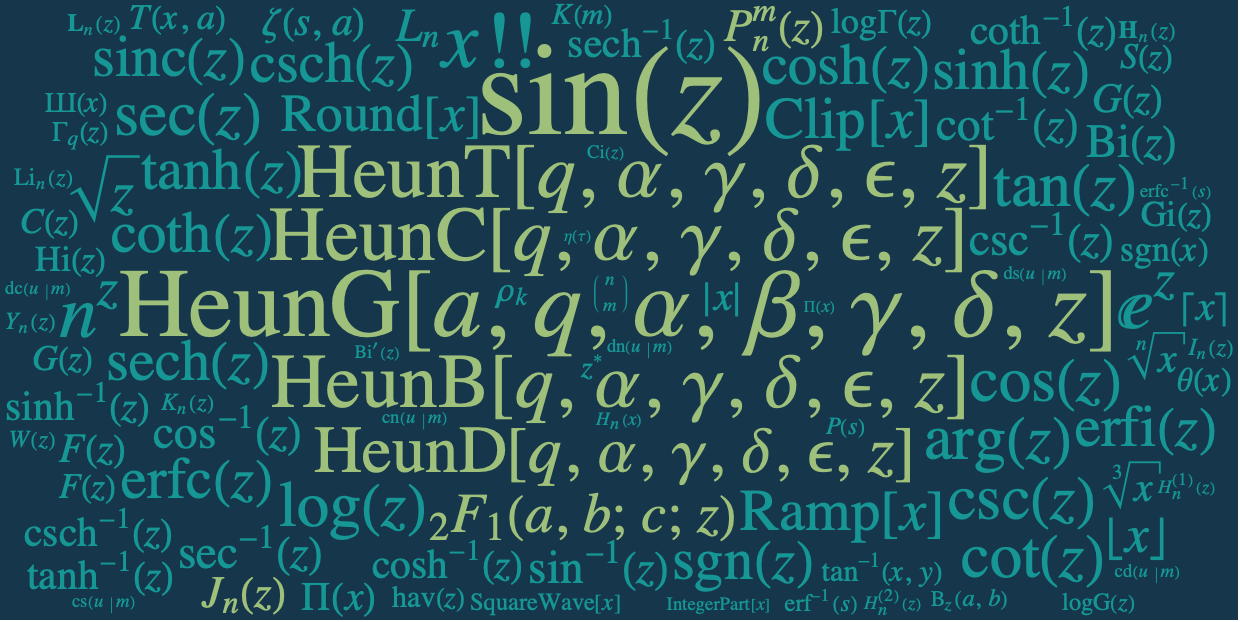My name is Tigran Ishkhanyan, and I am a special functions specialist in the Algorithms R&D department at Wolfram Research, working on general problems of the theory and advanced methods of special functions. I joined Wolfram at the beginning of 2018 when I was working on my PhD project in mathematical physics at the University of Burgundy, France, and at the Institute for Physical Research, Armenia.

My PhD project had two major directions: improvement of the theory of Heun functions and their application in quantum mechanics, specifically in the problems of quantum control in two-level systems and relativistic/nonrelativistic wave equations. I came up with the idea of implementing Heun functions into the Wolfram Language when I found out that this functionality had not yet been introduced.

## Elementary Functions

Every high-school student is familiar with simple functions such as Exp, Log, Sin and others—the so-called elementary functions. These functions are well studied and we know all their properties, but from time to time we are able to implement into the Wolfram Language something completely new and insightful like the ComplexPlot3D function that might be useful for educational and scientific purposes.

For example, here is the familiar sinusoidal plot for Sin: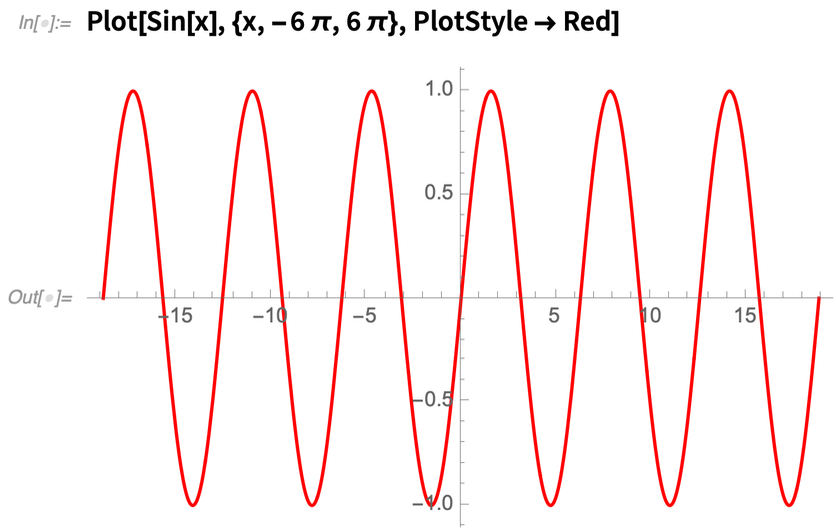✕ `Plot[Sin[x], {x, -6 \[Pi], 6 \[Pi]}, PlotStyle -> Red]`

And here is a Plot of the same function in the complex plane: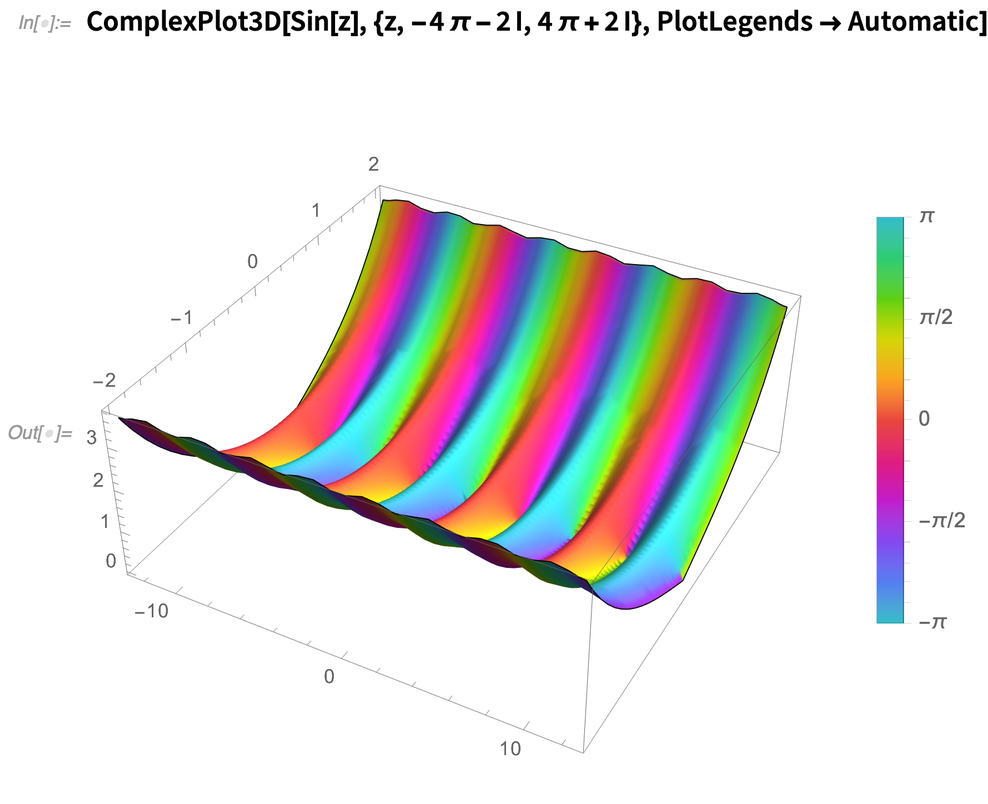✕ ```ComplexPlot3D[Sin[z], {z, -4 \[Pi] - 2 I, 4 \[Pi] + 2 I}, PlotLegends -> Automatic]```

## Special Functions

The special functions group is another subset of mathematical functions coming after the elementary ones. Special functions have been widely used in mathematical physics and related problems during the last few centuries. For example, the Bessel functions that describe the Fraunhofer diffraction and many other phenomena are special functions. In particular, the oscillatory behavior of BesselJ makes it suitable for modeling the oscillations of drums: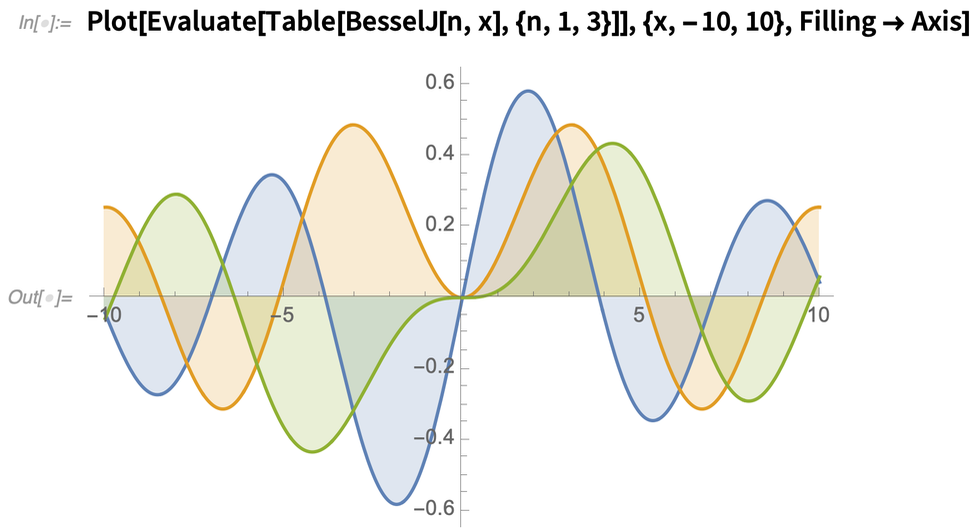✕ ```Plot[Evaluate[Table[BesselJ[n, x], {n, 1, 3}]], {x, -10, 10}, Filling -> Axis]```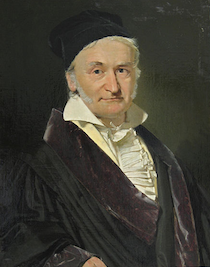In general, the Bessel-type functions, orthogonal polynomials and others are grouped in the class of hypergeometric functions: they are particular or limiting cases of different hypergeometric functions. The class of hypergeometric functions has a well-defined hierarchy, with the Hypergeometric2F1 and HypergeometricPFQ functions standing at the top of this class. The systematic treatment of these functions was first given by Carl Friedrich Gauss.

From the mathematical point of view, the general theory of hypergeometric functions is well developed. These functions had a significant impact in science (please explore the documentation pages of the hypergeometric functions for examples of applications).

There is also a group of advanced special functions. The Mathieu, spheroidal, Lamé and Heun functions are more general than the Hypergeometric2F1 function, so they are potent enough to solve more complex physical problems like the Schrödinger equation with a periodic potential: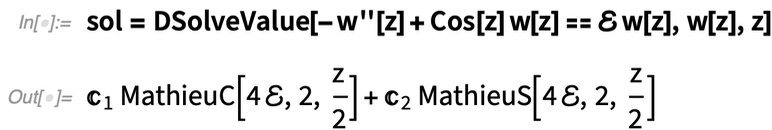✕ ```sol = DSolveValue[-w''[z] + Cos[z] w[z] == ℰ w[z], w[z], z]```

We have the Mathieu and spheroidal functions in the Wolfram Language, but what we didn’t yet have was the class of Heun (and as a particular case, the Lamé, or ellipsoidal, spherical harmonics) functions. We have implemented the missing group of Heun functions to achieve greater completion in covering the area of named special functions, as most of them are either particular or limiting cases of Heun functions. Its rising popularity in the literature indicates that the Heun class of functions is probably the next generation of special functions that will serve as a framework for future scientific developments. (For some nice references, please check the bibliography section of the Heun Project.)

## Development Perspective

There are two major directions of development for mathematical functions in the Wolfram Language: improved documentation for the functionality that is already in the system and implementation of new features, including new functions, methods and techniques of calculations.

In the first direction, we have recently standardized and significantly improved the documentation pages for the 250+ mathematical functions based on a large collection of more than 5,000 examples so that documentation pages now look like small, well-structured handbooks: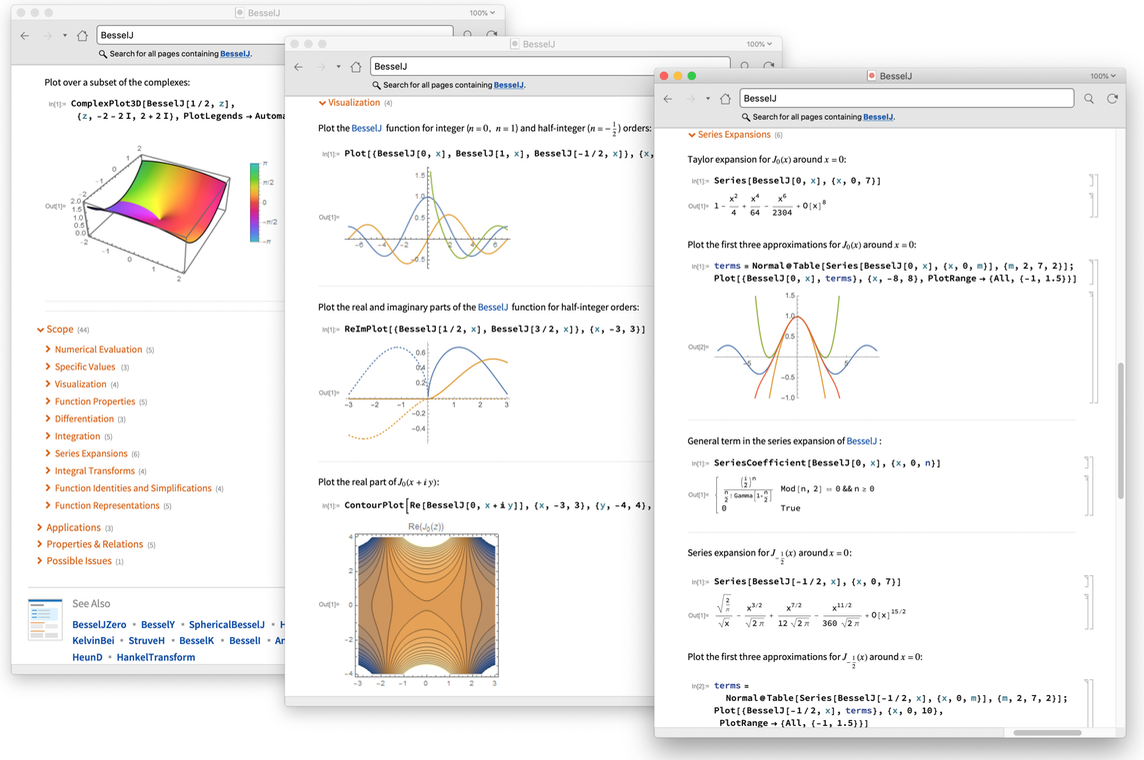In the direction of introducing new features, we have implemented powerful asymptotic tools like Asymptotic, AsymptoticDSolveValue and AsymptoticIntegrate. For Version 12.1, we have introduced 10 new Heun functions that are the most general special functions at the moment.

I will take a short detour and discuss the relation between mathematical functions and differential equations, since this provides the foundation for my approach to the Heun and other special functions.

## Functions Generated by Differential Equations

Many classical elementary and special functions are particular solutions of differential equations. Indeed, many of these functions were first introduced in an attempt to solve differential equations that arose in physics, astronomy and other fields. Thus, they may be viewed as being generated by the associated differential equations.

For example, the exponential function is generated by a simple first-order differential equation: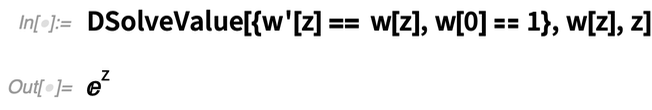✕ `DSolveValue[{w'[z] == w[z], w == 1}, w[z], z]`

Similarly, the following linear second-order differential equation generates the Legendre polynomials: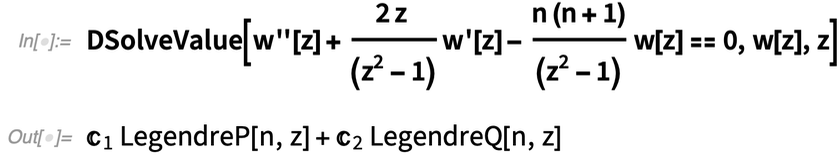✕ ```DSolveValue[ w''[z] + (2 z )/(z^2 - 1) w'[z] - (n (n + 1))/(z^2 - 1) w[z] == 0, w[z], z]```

I am a big fan of the idea of working directly with the differential equations instead of their particular solutions; this approach is much more beneficial, as differential equations are considered to be large data structures, and we are able to mine a lot of additional information about mathematical functions from their generating differential equations.

Now, the classification of linear differential equations is tightly connected with the structure of their singularities or singular points that might either be regular or irregular: these are the points in the complex plane where the coefficients of the differential equations diverge.

For the famous Bessel differential equation: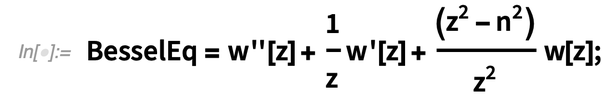✕ `BesselEq = w''[z] + 1/z w'[z] + (z^2 - n^2)/z^2 w[z];`

… that defines the Bessel functions: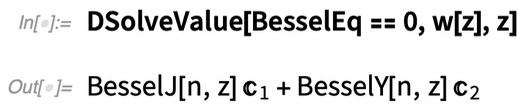✕ `DSolveValue[BesselEq == 0, w[z], z]`

… the point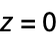is a regular singular point.

We may generate the solution of a linear differential equation at regular singular points using the Frobenius method, i.e. the power-series method that generates infinite-term expansions with coefficients that obey recurrence relations uniquely defined by the differential equation. The powerful AsymptoticDSolveValue function gives exactly these Frobenius solutions: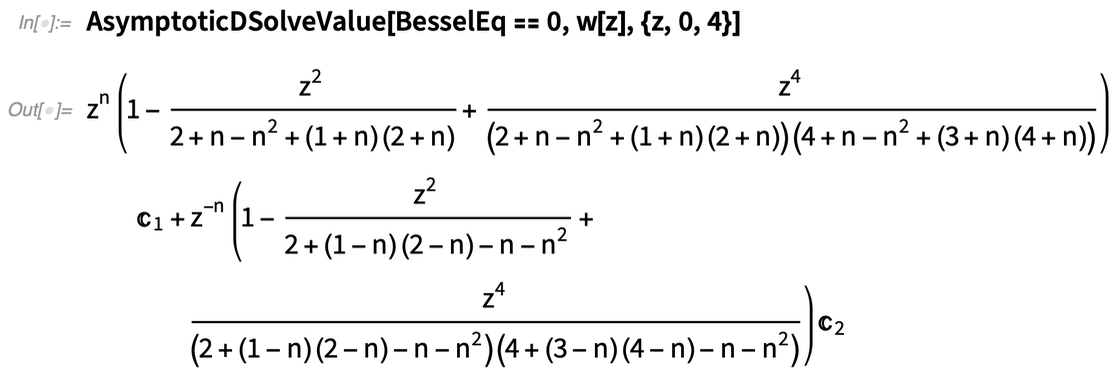✕ `AsymptoticDSolveValue[BesselEq == 0, w[z], {z, 0, 4}]`

Here the first Frobenius solution (the regular one at the singular point) is called BesselJ while the second one (the singular one) is called BesselY. Interestingly, this is a rather common situation in the theory of special functions. Of course there are exceptions to this rule, but usually special functions are Frobenius solutions of their generating equations at some regular singular points. For the Gauss hypergeometric equation that is the most general differential equation with three regular singular points located at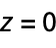,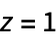and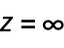: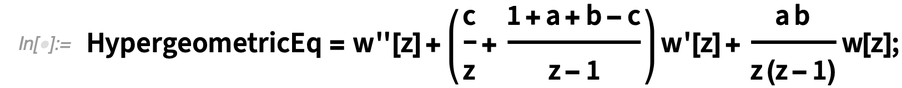✕ ```HypergeometricEq = w''[z] + (c/z + (1 + a + b - c)/(z - 1)) w'[z] + (a b)/(z (z - 1)) w[z];```

One of these Frobenius solutions (the regular one) is called Hypergeometric2F1 and is one of the most famous functions in physics: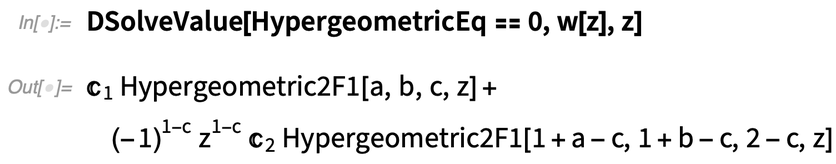✕ `DSolveValue[HypergeometricEq == 0, w[z], z]`

Naturally, the second solution in this output (i.e. the singular one with the pre-factor power function) is the second Frobenius solution of the Gauss hypergeometric equation.

The Hypergeometric2F1 function is an infinite series; the coefficients of this series obey a two-term recurrence relation of the form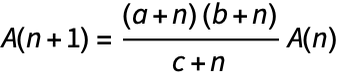: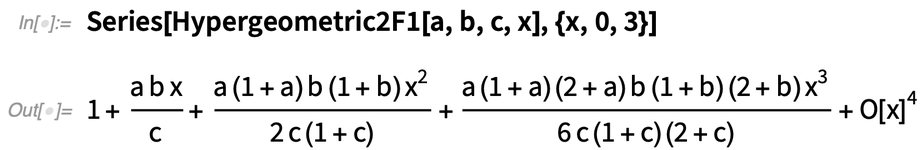✕ `Series[Hypergeometric2F1[a, b, c, x], {x, 0, 3}]`

… and there is an exact closed-form expression for the nth coefficient of the expansion. This is a common feature for all the hypergeometric functions.

But an important remark is that for advanced special functions (like the Heun functions), the coefficients of their Frobenius expansions obey recurrence relations of at least three terms. There are no general closed-form expressions for these functions. We do not know their explicit forms and obviously are forced to work with their generating equations that have one more singular point. This additional regular singular point leads to a significant complication of the solutions.

At last, after this brief diversion into the theory of special functions, we are ready to proceed and present the Heun functions.

## Heun Functions

Heun’s general differential equation is a second-order linear ordinary differential equation with four regular singular points located at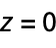,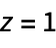,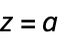and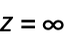on the complex plane: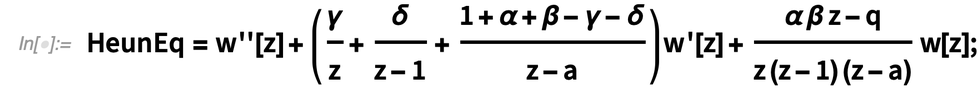✕ ```HeunEq = w''[ z] + (\[Gamma]/z + \[Delta]/(z - 1) + ( 1 + \[Alpha] + \[Beta] - \[Gamma] - \[Delta])/(z - a)) w'[ z] + (\[Alpha] \[Beta] z - q)/(z (z - 1) (z - a)) w[z];```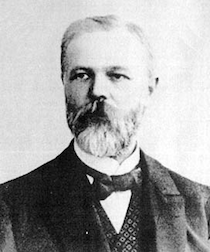The general Heun equation is a generalization of the Gauss hypergeometric equation with one more additional regular singular point located at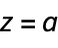(which is complex), so this equation is a direct generalization of the hypergeometric one with just one more regular singular point. This equation was first written in 1889 by Karl Heun, who was a German mathematician.

There is only one book and one chapter in the Digital Library of Mathematical Functions, plus around three hundred articles on different properties and applications of these general special functions. The theory of Heun functions is poorly developed, and a lot of important questions are still open but are being actively investigated.

The general Heun equation has six parameters. Four of them (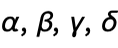) are the characteristic exponents of Frobenius solutions at different singular points: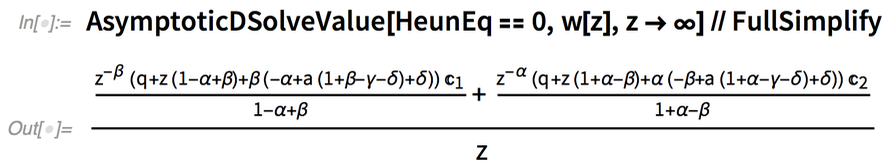✕ ```AsymptoticDSolveValue[HeunEq == 0, w[z], z -> ∞] // FullSimplify```

The parameter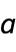stands for the third regular singular point, while the parameter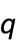—referred to as an accessory or spectral parameter—is an extremely important parameter that is not available in the case of hypergeometric functions.

In analogy with the hypergeometric equation, the regular Frobenius solution of the general Heun equation at a regular singular origin is called HeunG. It has the value of 1 at the origin and branch-cut discontinuities in the complex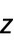plane running from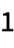to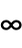and from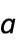to DirectedInfinity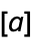: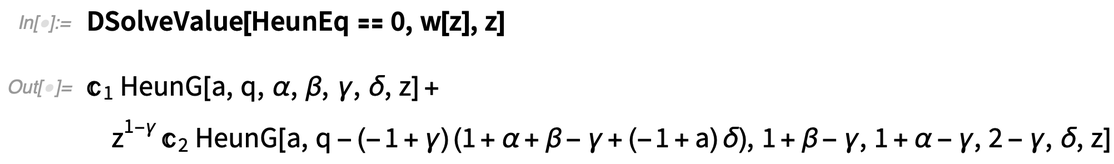✕ `DSolveValue[HeunEq == 0, w[z], z]`

The following shows a plot of the Heun functions for a range of values for the parameter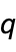: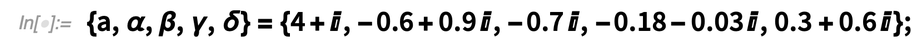✕ ```{a, \[Alpha], \[Beta], \[Gamma], \[Delta]} = {4 + I, -0.6 + 0.9 I, -0.7 I, -0.18 - 0.03 I, 0.3 + 0.6 I};```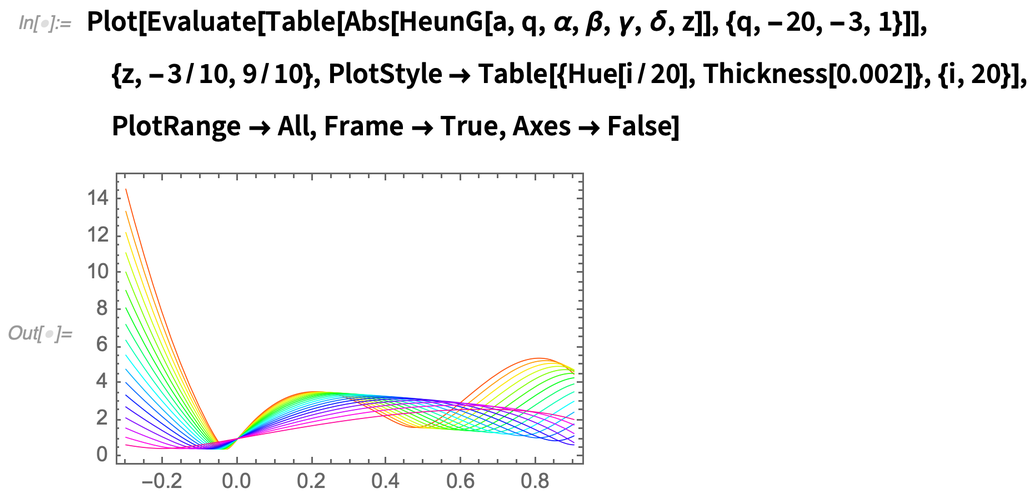✕ ```Plot[Evaluate[ Table[Abs[ HeunG[a, q, \[Alpha], \[Beta], \[Gamma], \[Delta], z]], {q, -20, -3, 1}]], {z, -3/10, 9/10}, PlotStyle -> Table[{Hue[i/20], Thickness[0.002]}, {i, 20}], PlotRange -> All, Frame -> True, Axes -> False]```

HeunG is simplified to Hypergeometric2F1 for the following sets of the parameters: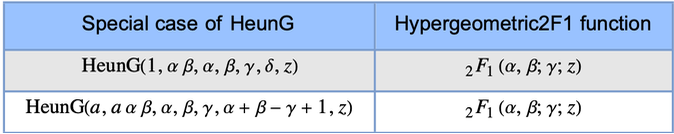A small but important remark here is that, although the closed forms of the Heun functions are unknown, different features of these functions might be revealed from the differential equations. For example, the transformation group of the HeunG function has 192 members (in total, 192 different local solutions for the general Heun equation, written in terms of a single HeunG function).

Unlike the hypergeometric functions whose derivatives are hypergeometric functions with shifted parameters, the derivatives of the Heun functions are special functions of a more complex class solving more complex differential equations. These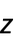derivatives were implemented as separate functions in Version 12.1. The derivative of HeunG is HeunGPrime: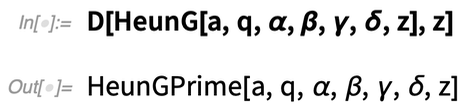✕ `D[HeunG[a, q, \[Alpha], \[Beta], \[Gamma], \[Delta], z], z]`

This pair of functions can be used to calculate the higher derivatives of HeunG using the differential equation to eliminate derivatives of order higher than one: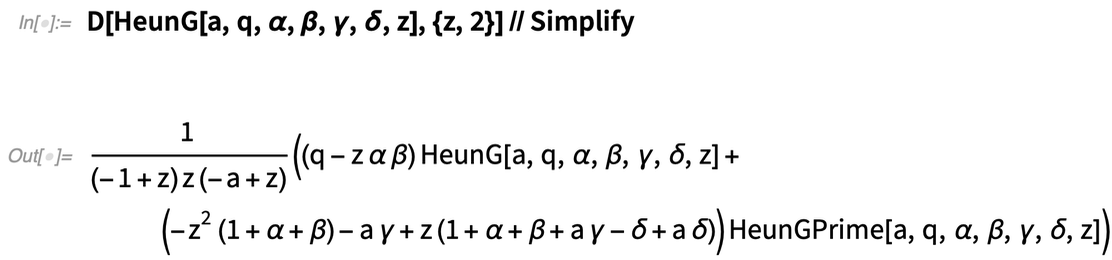✕ ```D[HeunG[a, q, \[Alpha], \[Beta], \[Gamma], \[Delta], z], {z, 2}] // Simplify```

Another feature is that indefinite integrals of Heun functions cannot be expressed in terms of elementary or other special functions: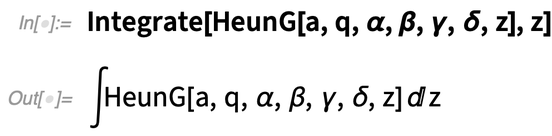✕ `Integrate[HeunG[a, q, \[Alpha], \[Beta], \[Gamma], \[Delta], z], z]`

Like the Hypergeometric2F1 function, HeunG has confluent cases when one or more of the regular singular points in the general Heun equation coalesce, generating equations with a different structure of singularities. We recall that Hypergeometric2F1 has one confluent case: the Hypergeometric1F1 function. HeunG has four confluent modifications called HeunC, HeunD, HeunB and HeunT solving the single-, double-, bi- and tri-confluent Heun equations, respectively.

HeunC has an invaluable importance as it generalizes the MathieuC and MathieuS functions, as well as others like the BesselI and Hypergeometric2F1 functions: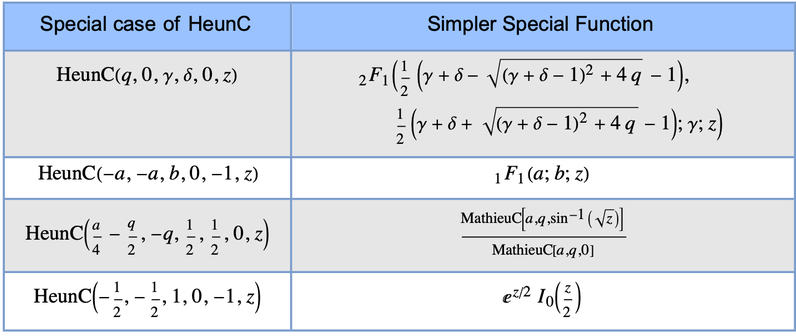A noteworthy example is that HeunC solves the generalized spheroidal equation in its general form without specification of the parameter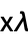: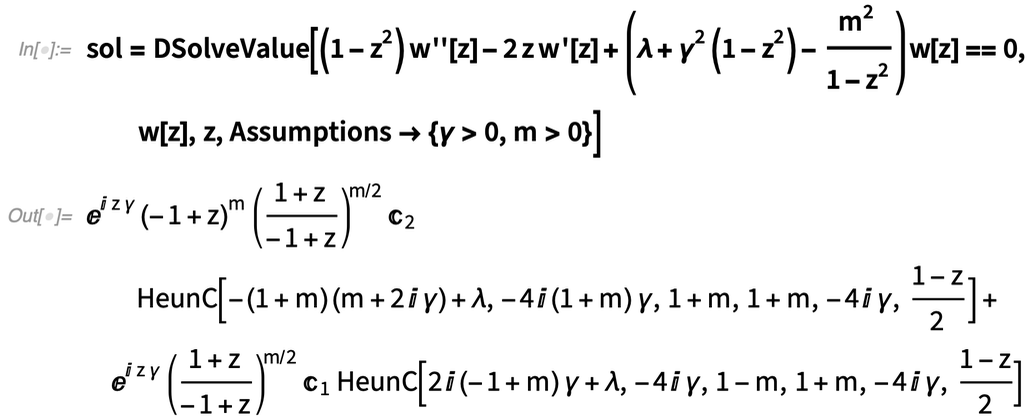✕ ```sol = DSolveValue[(1 - z^2) w''[z] - 2 z w'[z] + (\[Lambda] + \[Gamma]^2 (1 - z^2) - m^2/(1 - z^2)) w[ z] == 0, w[z], z, Assumptions -> {\[Gamma] > 0, m > 0}]```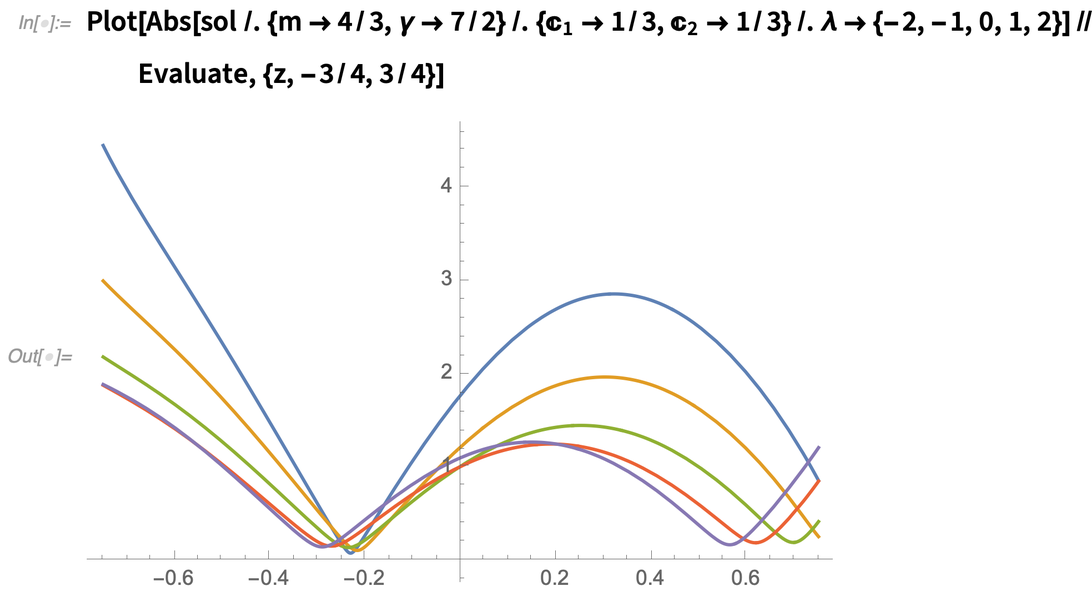✕ ```Plot[Abs[sol /. {m -> 4/3, \[Gamma] -> 7/2} /. {C -> 1/3, C -> 1/3} /. \[Lambda] -> {-2, -1, 0, 1, 2}] // Evaluate, {z, -3/4, 3/4}]```

HeunD is the standard series solution of the double-confluent Heun equation at the ordinary point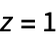: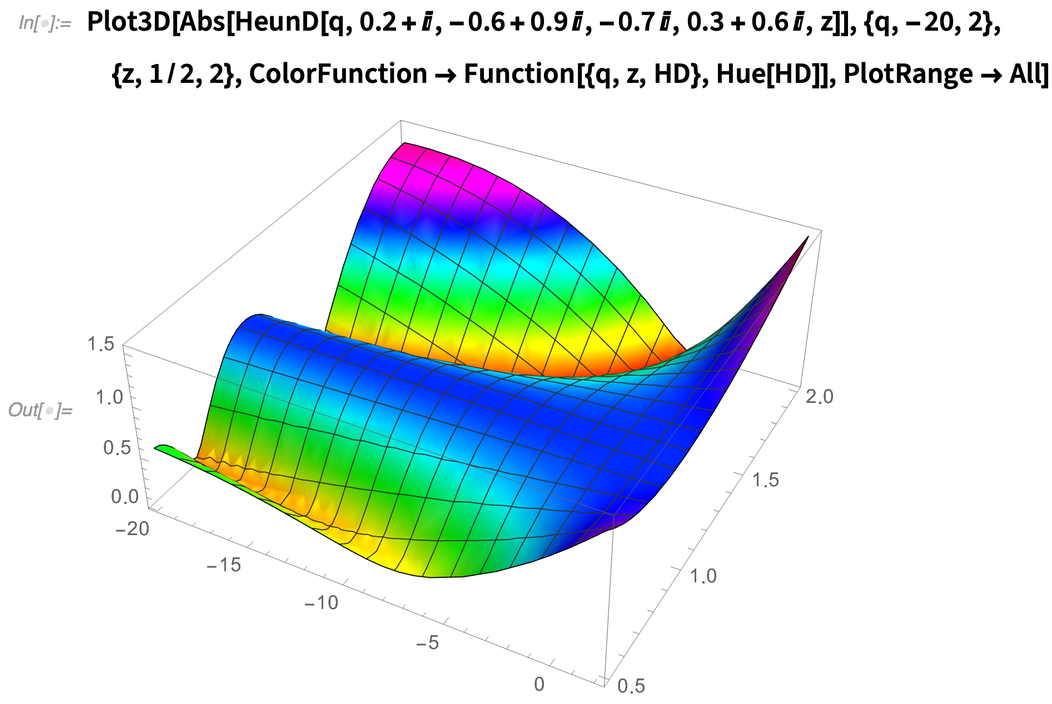✕ ```Plot3D[Abs[ HeunD[q, 0.2 + I, -0.6 + 0.9 I, -0.7 I, 0.3 + 0.6 I, z]], {q, -20, 2}, {z, 1/2, 2}, ColorFunction -> Function[{q, z, HD}, Hue[HD]], PlotRange -> All]```

The HeunB function solves the bi-confluent Heun equation: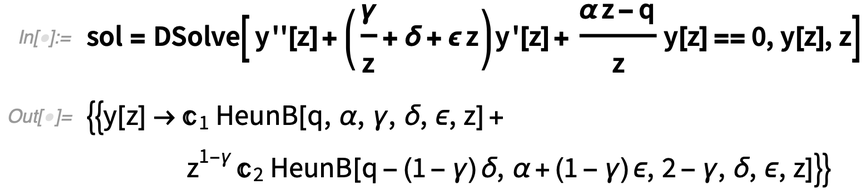✕ ```sol = DSolve[ y''[z] + (\[Gamma]/z + \[Delta] + \[Epsilon] z) y'[ z] + (\[Alpha] z - q)/z y[z] == 0, y[z], z]```

It has the following approximations around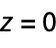: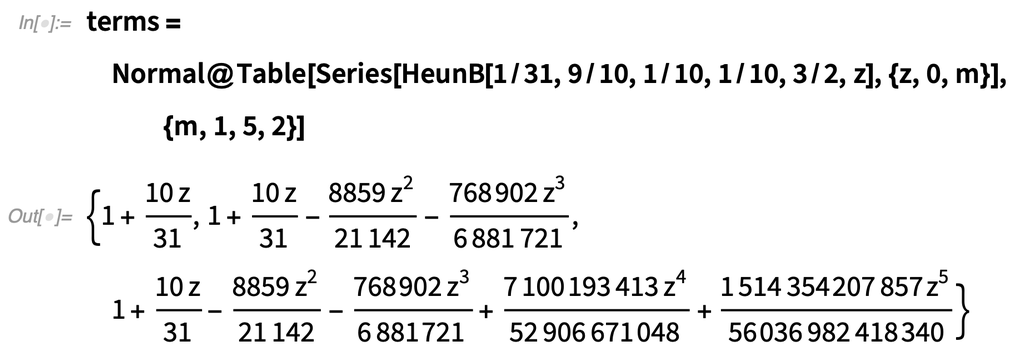✕ `terms=Normal@Table[Series[HeunB[1/31,9/10,1/10,1/10,3/2,z],{z,0,m}],{m,1,5,2}]`

Here is a plot of the approximations: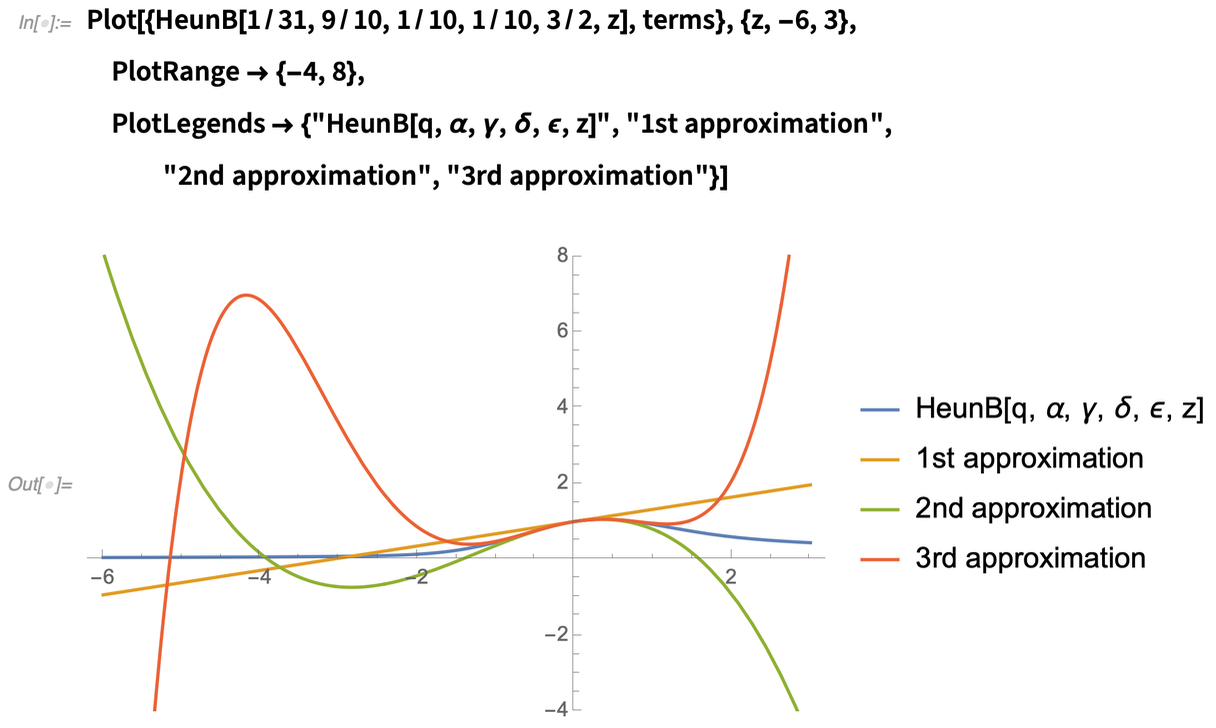✕ ```Plot[{HeunB[1/31, 9/10, 1/10, 1/10, 3/2, z], terms}, {z, -6, 3}, PlotRange -> {-4, 8}, PlotLegends -> {"HeunB[q, \[Alpha], \[Gamma], \[Delta], \[Epsilon], \ z]", "1st approximation", "2nd approximation", "3rd approximation"}]```

HeunB is truly useful, as different problems of classical and quantum physics are solved using this function. For example, the whole family of doubly anharmonic oscillator potentials (or, in fact, an arbitrary potential up to sixth-order polynomial form):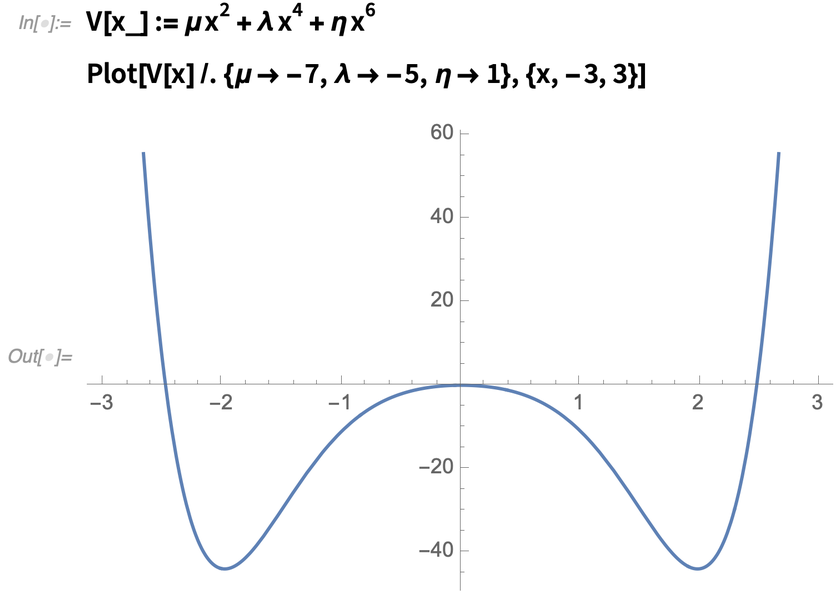✕ ```V[x_] := \[Mu] x^2 + \[Lambda] x^4 + \[Eta] x^6 Plot[V[x] /. {\[Mu] -> -7, \[Lambda] -> -5, \[Eta] -> 1}, {x, -3, 3}]```

… is solved in terms of the HeunB function: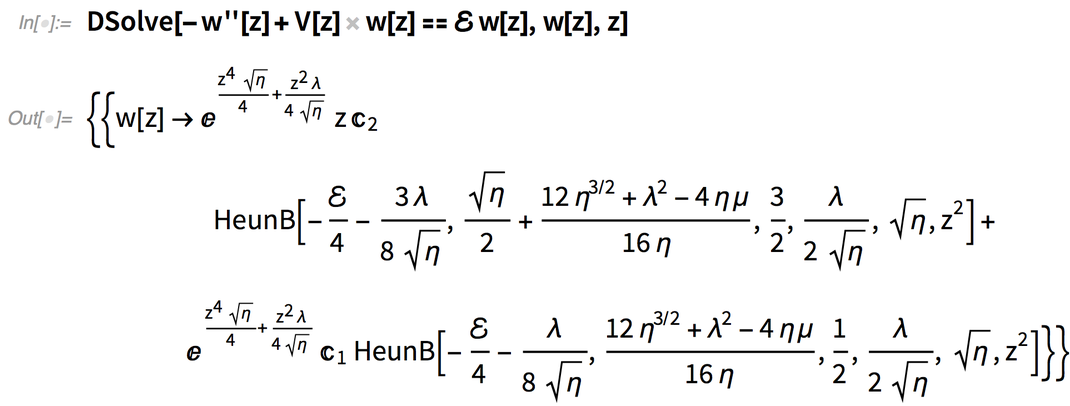✕ `DSolve[-w ''[z] + V[z] w[z] == ℰ w[z], w[z], z]`

… while the problem of normalizable bound states is still unsolved.

The last confluent Heun function, the HeunT function, which might be considered as a generalization of the Airy functions, is the solution of the tri-confluent Heun equation: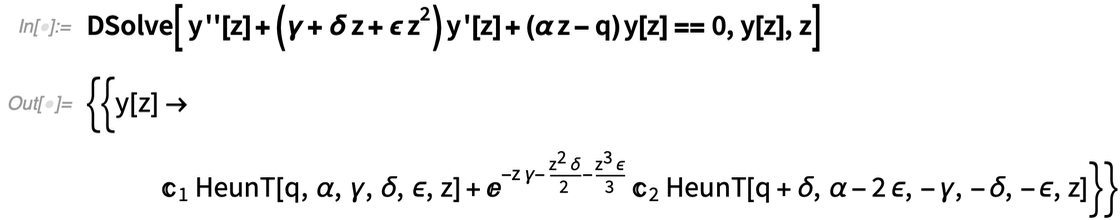✕ ```DSolve[ y''[ z] + (\[Gamma] + \[Delta] z + \[Epsilon] z^2) y'[ z] + (\[Alpha] z - q) y[z] == 0, y[z], z]```

HeunT solves the classical anharmonic oscillator problem (in fact, the quartic potential):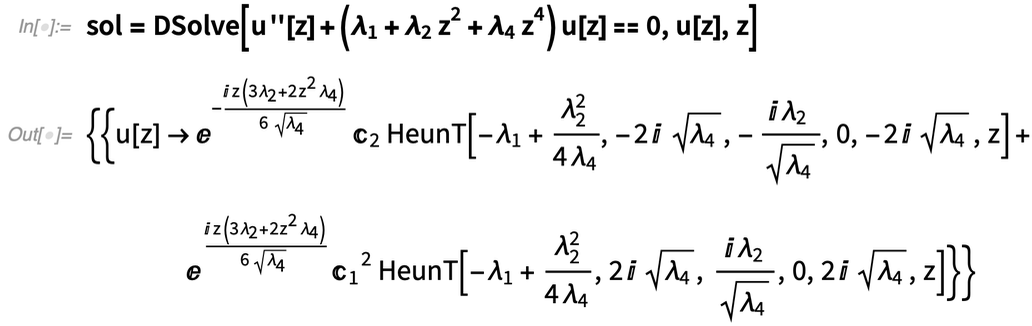✕ ```sol = DSolve[ u''[z] + (Subscript[\[Lambda], 1] + Subscript[\[Lambda], 2] z^2 + Subscript[\[Lambda], 4] z^4) u[z] == 0, u[z], z]```

We are able to simulate the dynamics of the oscillator using HeunT functions: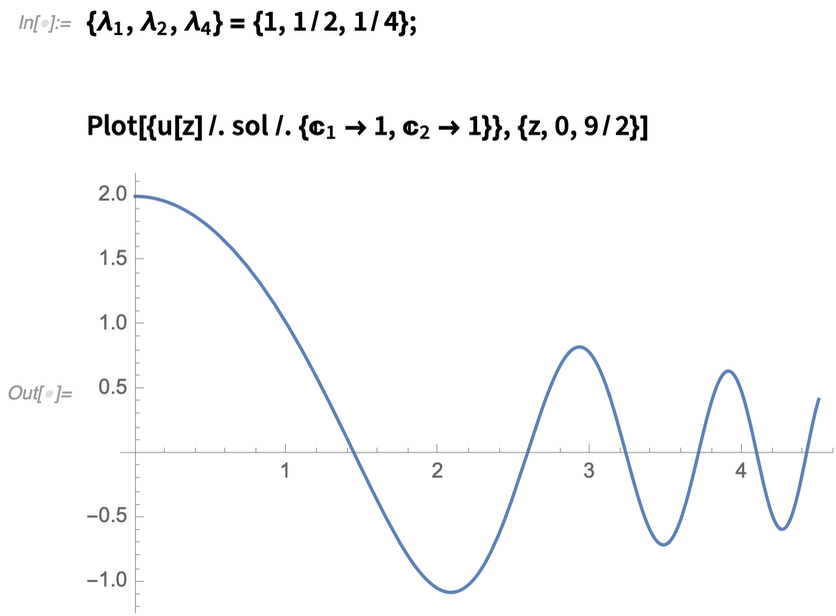✕ ```{Subscript[\[Lambda], 1], Subscript[\[Lambda], 2], Subscript[\[Lambda], 4]} = {1, 1/2, 1/4}; Plot[{u[z] /. sol /. {C -> 1, C -> 1}}, {z, 0, 9/2}]```

Surprisingly (or not?) the “primes” of the Heun functions are independent actors and have important applications in science.

The Wolfram Language also has the MeijerG superfunction, with a powerful tool set and wide variety of features: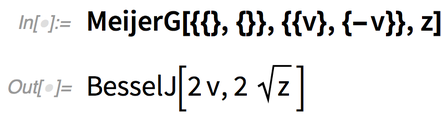✕ `MeijerG[{{}, {}}, {{v}, {-v}}, z]`

Unfortunately, the MeijerG representations of special functions are limited to the hypergeometric class of functions and are not applicable in the Heun case (as well as Mathieu and spheroidal cases).

These and a lot of other interesting examples on the properties and applications of the Heun functions are noted in the documentation pages.

## Heun Functions in Physics

Heun functions have a range of applications in contemporary physics and are powerful enough to generate solutions for a significant set of unsolved problems from quantum mechanics, the theory of black holes, conformal field theory and others. They are being successfully applied in real physical problems at a rapid rate: during the last decade, the number of publications related to the theory of Heun functions tripled in comparison with all other publications until 2010, according to arXiv.

Specifically, the powerful apparatus of the Heun functions allows derivation of new infinite classes of integrable potentials for relativistic and nonrelativistic wave equations used in different problems of quantum control and engineering (please see the recent paper by A. M. Ishkhanyan for different examples).

Heun functions appear in the theory of Kerr–de Sitter black holes and may be used for analysis in more complex geometries (the papers by R. S. Borissov and P. P. Fiziev and H. Suzuki, E. Takasugi and H. Umetsu discuss these problems).

The relationship between the Heun class of equations and Painlevé transcendents leads to new results for the two-dimensional conformal field theory based on the analysis of the solutions of Heun equations (see the papers of B. C. da Cunha and J. P. Cavalcante and F. Atai and E. Langmann).

The aforementioned examples as well as others indicate that the Heun functions are important in and popular for solving absolutely different problems in contemporary physics.

## Closing Words

At Wolfram, we are in a constant search for fresh ideas and methods that make the Wolfram Language one of the most famous, popular, powerful and user-friendly tools for scientists working in different areas of contemporary science.

From time to time, the mathematical toolset has to be updated to meet new problems and challenges. Twentieth-century quantum mechanics is closely related to the hypergeometric class of functions, but the set of problems solvable with these special functions is largely exhausted, so a new generation of functions is needed. This is why for Version 12.1 of the Wolfram Language, we implemented the Heun functions and plan to continually improve the coverage of advanced special functions to meet more complex scientific challenges in the future.

 Get full access to the latest Wolfram Language functionality with a Mathematica 12.1 or Wolfram|One trial.